# Fitzsimmons-Fristedt-Shepp theorem

A theorem asserting that two particular random sets obtained in quite different ways have the same distribution law [a1]. A first version of this theorem was obtained by B. Mandelbrot in 1972 [a2]. It is a key fact for understanding the link between random coverings and potential theory (see also Dvoretzky problem; Billard method).

The first random set is defined asHere, the random cutoutsare associated with pointsthat are randomly distributed in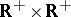in such a way that their number in any given rectangle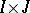is a Poisson random variable (cf. Poisson process) with parameter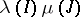, whereis the Lebesgue measure andis a given measure on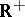that is locally bounded except at. The set of pointsis called a point Poisson process with intensity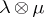, andcan be viewed as the set of points inthat are never in the shadow of the point Poisson process when light comes from the directions,.

The second random set is the closure of the range of a positive Lévy process with driftand Lévy measure. By definition, this process,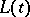(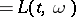), has independent and stationary increments, and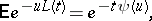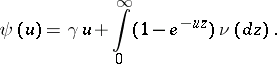The theorem asserts that for any givenwith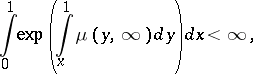(a1)

one can explicitly defineandin such a way that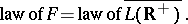The driftvanishes precisely when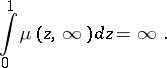For example, when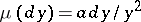,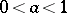, then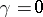and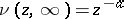(this is the case of a stable Lévy process of index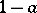).

When the integral in (a1) is infinite, a formal computation givesandconcentrated at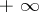. This is the case whenis empty. Therefore, the Fitzsimmons–Fristedt–Shepp theorem is an extension of Shepp's theorem, which states that (a1) is a necessary and sufficient condition for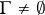almost surely [a3].

Now, given a compact subsetof, the probabilities of the eventsandare the same; in other words:almost surely if and only ifis a polar set for the Lévy process. Since compact polar sets are precisely the compact sets of vanishing capacity with respect to a potential kernel (associated withandand therefore with), the link between Poisson covering ofand potential theory is manifest (see also Billard method).

How to Cite This Entry:
Fitzsimmons-Fristedt-Shepp theorem. Encyclopedia of Mathematics. URL: http://encyclopediaofmath.org/index.php?title=Fitzsimmons-Fristedt-Shepp_theorem&oldid=22429
This article was adapted from an original article by J.-P. Kahane (originator), which appeared in Encyclopedia of Mathematics - ISBN 1402006098. See original article